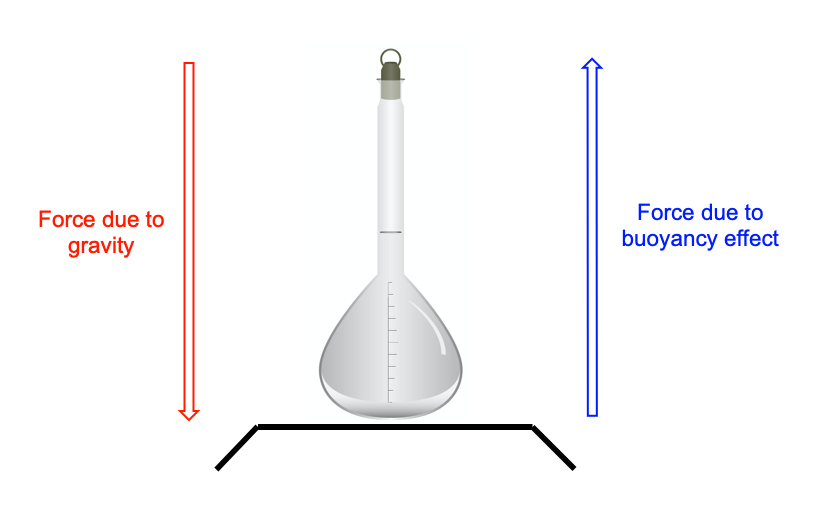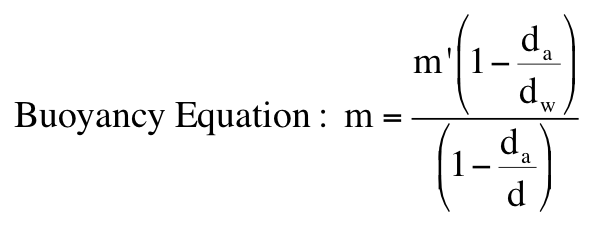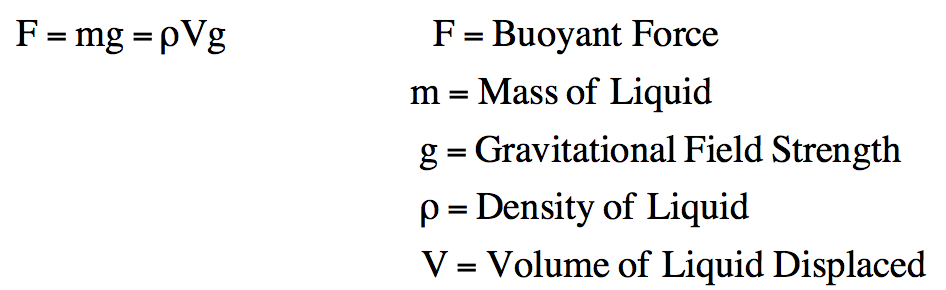Clutch Prep is now a part of Pearson
Ch.2 - Tools of the TradeWorksheetSee all chapters

# Buoyancy

See all sections
Sections
Safety & Labels
Buoyancy
Thermal Dependency
Volumetric Instruments
Filtration & Evaporation

Buoyancy represents the upward force an object either by a liquid or air.

###### Buoyancy in Air

Example #1: When weighing an object you must take in to account the two opposing forces acting upon the object.In order to determine the true mass of an object you utilize the buoyancy equationExample #2: A convenient method in calibrating pipets is to weigh the water delivered from them. By using the density of water at a given temperature we can determine the volume of delivery with greater accuracy. Assume a 50.0 mL pipet is in need of calibration. An empty flask weighs 49.563 g. When water delivered from the pipet is added to the empty flask the new mass is recorded as 69.618 g. What is the mass of water delivered? The density of the standard weights is determined to be 8.40 g/mL.

###### Buoyancy in Fluids

Example #3: When fully or partially submerged in a fluid, the buoyancy force is directly proportional to the weight of the liquid displaced.Example #4: A wooden block with measurements of 0.15 x 0.44 x 0.56 m is afloat on a lake. If it is submerged by 0.031 m, what is its mass?

###### Buoyancy Calculations

Example #5: A small crystal of sucrose (C12H22O11) had a mass of 5.345 mg. The dimensions of the box-like crystal were 2.20 mm x 1.36 mm x 1.12 mm. What is the density of the sucrose crystal expressed in g/mL?

Practice: An empty container weighing 73.190 g is filled with an unknown liquid and the combined mass is recorded as 87.308 g. The container was then emptied and filled with water and recorded a new mass of 88.442 g at a temperature of 19 oC. (d = 1.0027 g/mL). Calculate the density of the unknown liquid.

Example #6: A piece of concrete weighs 120 N. When it is fully submerged, its apparent weight is 97 N. Determine the density of the water if the volume of the water displaced is 4200 cm3. (g = 9.8 N/kg)

Example #7: The density of propane, an odorless hydrocarbon compound used in cooking, is 0.922 g/mL. When a sample of it is placed on an analytical balance a weight of 8.15 x 108 ng is obtained. Calculate the true mass of propane.

Practice: An object weighs 36 g in air and has a volume of 8.0 cm3. What will be its apparent weight when immersed in water?